## 10 Questions MCQ Test Mathematical Olympiad Class 8 | Olympiad Test: Achieves Section-2

Description
Attempt Olympiad Test: Achieves Section-2 | 10 questions in 20 minutes | Mock test for Class 8 preparation | Free important questions MCQ to study Mathematical Olympiad Class 8 for Class 8 Exam | Download free PDF with solutions
QUESTION: 1

### What is the value of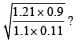Solution: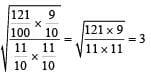QUESTION: 2

### 9x2 + 25 - 30x is the square of

Solution:

9x2 + 25 - 30x
= (3x)2 + (5)2 - 2 (3x) (5)
= (3x - 5)2

QUESTION: 3

### One of the factor of x2 + 17x + 60 is

Solution:

x2 + 17x + 60
=x2 +5x +12x + 60
=x(x+5) +12(x+5)
=(x+5) + (x+12)

QUESTION: 4

The perimeter of a rectangle is 54 cm. If its width is 2 cm more than one-fourth of its length. What is its length?

Solution:

Let the length be x.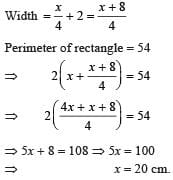QUESTION: 5

One of the letters from the word SOCIOLOGY is chosen at random. What is the probability that this letter is O?

Solution:

Total number of letters = 9
Number of letter ‘O’ = 3
Required probability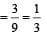QUESTION: 6

What is the value of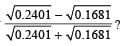Solution: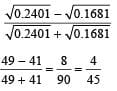QUESTION: 7

The product of two numbers is 1575 and their quotient is 9/7. What is the difference of the numbers?

Solution:

Let the numbers be x and y.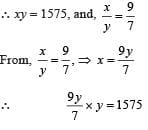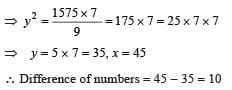QUESTION: 8

The diagonal of a square is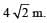What is its perimeter?

Solution: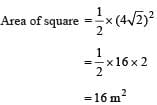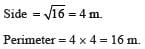QUESTION: 9

What is the simplified value of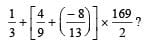Solution: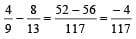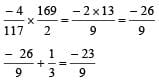QUESTION: 10

A well with internal diameter 8m is dug 7m deep. The earth taken out of it is spread at around to a width of 2 m to form an embankment. The height of embankment will be :

Solution:

Volume of earth dug out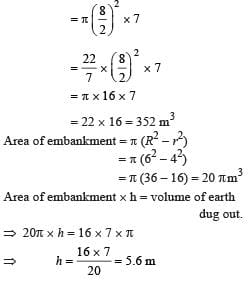Use Code STAYHOME200 and get INR 200 additional OFF Use Coupon Code